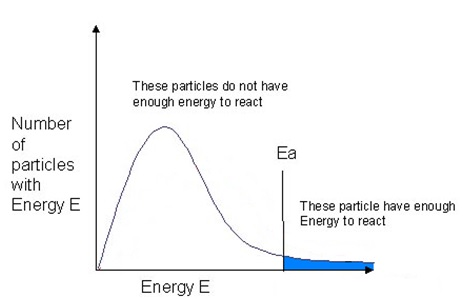Collision Theory and Temperature

As temperature increases, the rate of the reaction increases. In general, for every 10oC increase in temperature that occurs, the rate of the reaction doubles.

Temperature of an object measures the average kinetic energy that is found in that sample of matter, with some particles having very little kinetic energy and others with a high amount of kinetic energy. For gases, this can be shown on a graph called the Maxwell-Boltzmann Distribution which is a plot of the number of particles having each particular energy.

The area under the curve is a measure of the total number of particles present Figure 1.+For a reaction to occur, the particles must collide with energies equal to or greater than the activation energy for the reaction. We can mark the activation energy on the Maxwell-Boltzmann distribution (Figure 2). Notice that the large majority of the particles don't have enough energy to react when they collide.An increase in temperature results in increased energy for molecules, causing the particles to move more. This increase in energy shifts the Maxwell-Boltmann distribution to where more particles have higher energy (Figure 3). The total number of particle under the curve remains the same, as well there are still some particle with low energy. However, there are a higher proportion of molecules with energy above the activation energy (Figure 3). At the higher termperature (red line) there are more particles with energy above the threshold or activation energy. As a result there will be an increased proportion of particles that have sufficient energy for an effective collision.

Therefore, collision theory explains the affect of increasing temperature on the rate of a reaction by:

• at higher temperatures particles are moving more, this increased movement will increase the number of collision that can occur
• as temperature increases, the number of particles with sufficient energy for an effective collision will increase, resulting more of the collisions being effective.